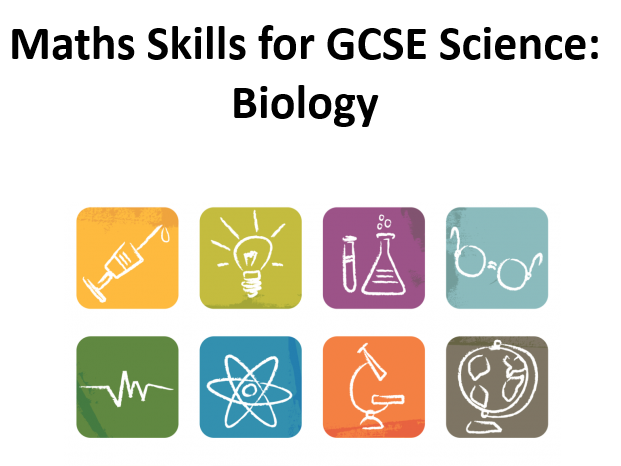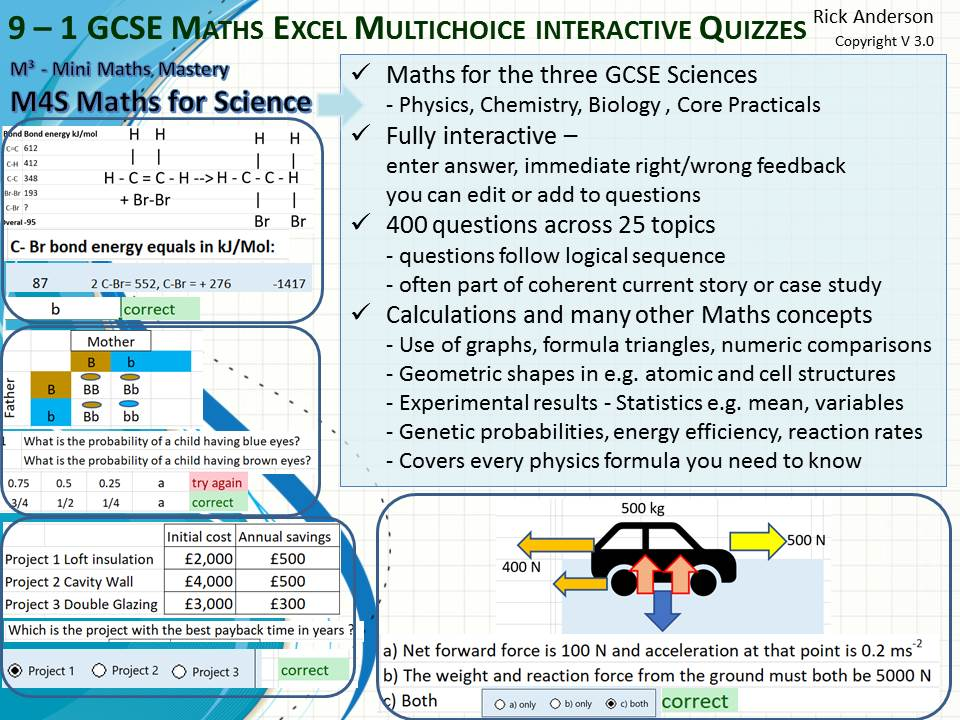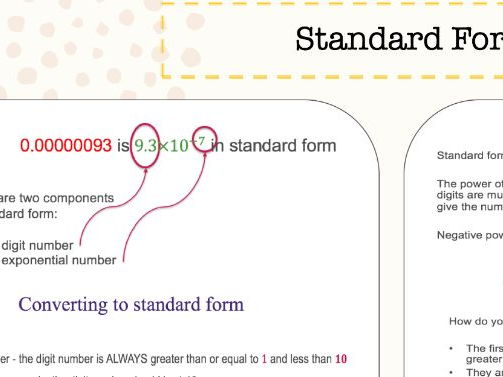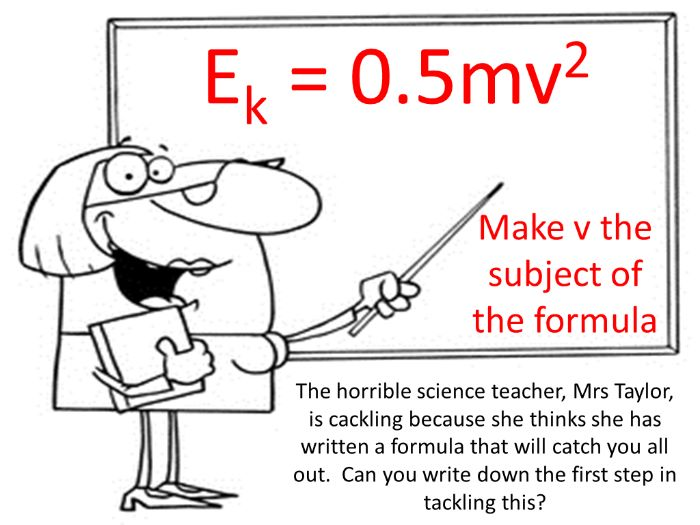# GCSE science: maths skills

Resources team
08th January 2020# GCSE and iGCSE maths skills resources for science

With maths skills taking a 1:2:3 weighting in both GCSE and iGCSE sciences, it can be tricky for students to get to grips with all of the materials. So, to help increase your students confidence with maths in biology, chemistry and physics, we have pulled together a range of resources to cover the basics and build on their knowledge.#### Maths Skills in Biology

Lesson on Maths skills needed for the new Combined Science GCSE (9-1) with a focus on Biology content, although many of the skills are needed across all three Sciences.

The lesson is intended to be a circus activity with all of the topics around the room (information sheet and exam question sheet for each that I printed back to back) for students to move around between and then mark at the end of the lesson. It could easily be done as a teacher lead lesson as well though and even slotted into other lessons as needed.

The maths skills included are:

• Comparing Data
• Analysing Graphs
• Drawing Tables
• Calculating the Mean
• Mode & Median
• Calculating Percentages
• Calculating Percentage Changes
• Percentile Charts
• Converting Units
• Standard Form
• Ratios & Fractions
• Variation
• Magnification Equation
• Cardiac Output Equation
• BMI Equation
• Estimating Population Size Equation

There is an information sheet and exam question sheet for each, as well as answers to all the questions.#### Use of Greek Letters in Maths (and Science) Display

All letters of the Greek alphabet, with a list of the most prominent uses in both mathematics and science. Perfect for displaying around the perimeter of a classroom.#### Maths in Science Posters

A set of 5 posters covering most of the key mathematical ideas covered in the KS4 syllabus. The posters can be printed out in a range of sizes to meet your needs.

Thanks for looking#### SAMPLE: New GCSE Maths Skills for Science: Biology

**This is a sample of the Maths Skills handbook I have for sale [https://www.tes.com/teaching-resource/new-gcse-maths-skills-for-science-biology-11884031] get a feeling of the content and the see the entire contents page to see all of the areas covered. The full resource is described below.
**

This resource is the culmination of many hours work, making sense of the new required Maths Skills for the 1-9 Science GCSE.

I was tasked to complete this, for my RPD and have made every effort to make clear explanations and give worked examples for every area of the Maths Skills requirements needed for GCSE Biologists from all specifications.

So far the feedback from our students has been great and they have found the wording to be clear and simple.

There are quick and simple questions the students can complete after each section as well as relevant past exam questions at the end of most chapters.

I believe this booklet will really help clarify some of the confusing areas up the Maths requirement for GCSE Biology.

Save yourself the time and effort of creating something similar for the price of a couple of coffees…#### Efficiency Calculations. 10 easy, 10 medium, 10 hard. Including Answers. UPDATED 19.07.16

This is part of Maths (The Physics Bits) for Science Students, available in my shop. Versions for each exam board available.

This is also available as part of a discount bundle, if you are interested in a number of my resources and want to talk about a custom discount, please get in contact

The easy questions are aimed at foundation tier C grade students; these do not require students to rearrange equations or convert non-standard units. The skills involved are identification of the correct numbers to use, correct use of a calculator and correctly method for answering questions.
The medium questions are aimed at B grade students; they involve rearranging equations as well as all the skills needed for the easy questions, there is some application of logic and the need for correct use of standard form and significant figures.
The hard questions are aimed at A and A* students; they not only require rearranging equations, but a wide variety of non-standard units that need to be converted, combining two or more equations and the application of logic, correct use of standard form and significant figures.
Each section has 5 simple calculations in a table format, and then five questions written in exam style. The language used is different in each question (find/calculate/work out/determine) to prepare student for the range of language they will come across in an exam. Each section of 5 written questions gets gradually harder as they go on, to challenge students and encourage them to move on to the next level.#### Maths for GCSE 9-1 Science 400 questions

Many good GCSE science students lose marks because they are worried by the maths but this 400 question multichoice quiz - see video - covering 30 theory and core practical topics across Chemistry, Physics and Biology - will help and features precisely the maths competencies I identified from extensive research into past papers.
Maths for Science covers obvious requirements like mean, interpreting graphs, rounding, percentage change and probability; but also extends for example to use of formula triangles and rearranging formulae, use of control, dependent and independent variables in experiments, chromosome counts, atomic mass and number, units of measure, isotope decay, carbon dioxide concentration. In fact anything that involves a number!
When you enter an answer by clicking, entering a,b,c or a number you get an immediate correct/try again colour coded answer. There is a reset button to start again. You can add questions with just a modicum of Excel skills.#### GCSE Maths skills in Science

Classroom display that teaches students about the maths skills required for GCSE science according to the new specification.

includes:
Standard form
orders of magnitude
significant figures
rounding numbers
estimates
averages
mean
mode
median
probability
algebra
simplifying

each topic is on a single slide which can be printed a3 (or a4). includes rules and worked examples of questions#### GCSE Sciences - Maths for Science Knowledge Organiser

This knowledge organiser is a great revision aid for all those maths skills that students need for the new GCSE Biology, Chemistry, Physics or Combined Science. It covers standard form, conversions, definitions of keywords such as uncertainty and precision, graphs and operator precedence.

Use it for students as a mat to aid in calculations or as a summary of knowledge to be learned.#### Rearranging the formula (Maths in Science)

An informative lesson presentation (37 slides) and accompanying worksheets that guides students through the different methods that can be used to rearrange formulae as they will be required to do in the Science exams. The lessons shows them how to use traditional Maths methods involving inverse operations and also equation triangles to come to the same result. These are constantly linked to actual examples and questions to show them how this has to be applied. There are regular progress checks, with explained answers, so that students can assess their understanding.#### Maths in science activities

12 maths in science activities that can be used for both KS3 and KS4
Each activity takes up a single slide so there is no need to have to flick forwards and backwards.
The activities require no additional printing and teacher guidance is included in the notes underneath each slide.
Students carry out activities such as the interpretation of data in a range of formats, using standard form, using equations.
These can be used as starters or as extended activities depending on the age and ability of students